Browse by Topic
Related Topics

# Arithmetic Is Hard—To Get Right

I’ve been working on arithmetic in Mathematica for more than 12 years. You might think that’s silly; after all, how hard can arithmetic be?

Today we were reminded again about how hard it can be. A nasty little bug in Excel 2007 came to light, whereby the result of computing, for example, 850*77.1 is displayed as 100000: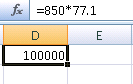Of course, this works just fine in Mathematica: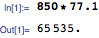But why is arithmetic so difficult to get right?

The standard “schoolbook” algorithms are pretty easy. But they’re inefficient and often unnecessarily inaccurate. So people like me have done a huge amount of work to find algorithms that are more efficient and accurate. And the problem is that these algorithms are inevitably more complicated, and one has to be very careful to avoid insidious bugs.

Take multiplying integers, for example. The standard “schoolbook” long-multiplication algorithm uses n^2 multiplications to multiply two n-digit numbers. But many of these multiplications are actually redundant, and we now know clever algorithms that take n^1.58, n log n or even fewer multiplications for large n. So this means that if one wants to do a million-digit multiplication, Mathematica can do it in a fraction of a second using these algorithms—while it would take at least a few minutes using standard long multiplication. The black squares below show the multiplications required for the schoolbook and Karatsuba algorithms respectively.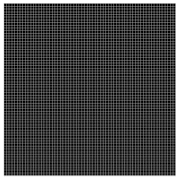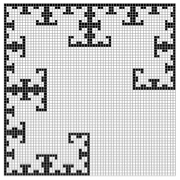Today’s Excel bug is actually not about underlying multiplication, but instead about displaying numbers in base 10. Current computers essentially all represent numbers in binary. But when one outputs them, one has to convert to base 10.

Doing this conversion might seem to be the “easy part.” But actually, it’s really subtle. The main issue is being able to round the underlying binary form to the “nearest representation” in decimal.

If one gets it wrong, very bizarre things can happen. For example, one can enter a number in decimal, have it converted to binary to be stored and then have it end up being a different number when one prints it out. And in fact, this happens in Excel, not only in the 2007 version but also in previous versions. Here one enters 40000.223 and it shows up as 40000.2229999999: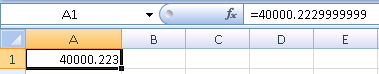Converting from binary to decimal is based on multiplication; converting from decimal to binary is based on division. The tricky part is that for some numbers, the multiplication or division needs to be done to a higher precision than the numbers themselves in order to get the correct answers.

Most computer systems just work with numbers at a fixed precision that’s immediately available from the underlying hardware. And if one can’t do anything to increase the precision, it’s simply not possible to always get the right answers for binary-to-decimal and decimal-to-binary conversions.

Mathematica takes a much more sophisticated view of numbers. Ever since the beginning, it’s allowed numbers to have arbitrary numbers of digits, and arbitrary precision—and it can track precision through a computation.

One might have thought that arbitrary precision would only be important for something esoteric like computational number theory. But in fact, what we’ve seen in recent years is that it’s increasingly crucial in ensuring reliability in everyday computations. So it’s really nice that it’s built in throughout the Mathematica system.

One of the strange things about arithmetic and arithmetic algorithms is that it can be fairly easy to get them almost right—but there can be weird bugs that crop up only in rare cases.

This has happened many times. The Pentium FDIV bug in 1994 occurred only for particular numbers that happened to sample an incorrect entry in a lookup table. The Ariane 5 rocket explosion in 1996 occurred because a conversion between number types failed for particular numbers. And so on.

Carries are a particularly common cause of rare bugs. What can happen is that there may be only some rare class of numbers for which a carry propagates in a particular way that causes trouble. Problems like this have a long history; Charles Babbage’s original mechanical computers in the 1820s would get stuck if they had to carry too far. And most likely the problem in Excel today is associated with carries.

One problem with rare bugs that afflict only numbers with particular patterns of bits is that they can be very hard to catch in testing. One can run an algorithm on billions of numbers, and never see a problem.

But if one is working on some particular computation that happens to generate numbers with a particular structure, one can fall right into the bug.

When we test Mathematica, we put a lot of effort into systematically testing all of the “fringe” cases where unusual things might happen. And doing this correctly requires quite sophisticated knowledge not only of testing but also of the underlying algorithms that are used.

These days reliability is an increasingly important component of numerical computation. Machines have become so fast that people are doing huge numbers of numerical computations all the time. And now what’s critical is to get them right all the time—because if there’s a fringe case that’s wrong, it’s now going to be noticed.

It’s not easy to get reliable numerical computation, and it’s not something one can “bolt on” after the fact. It’s something one has to build in from the beginning, as we’ve done in Mathematica for nearly 20 years.